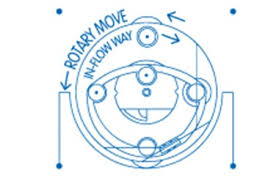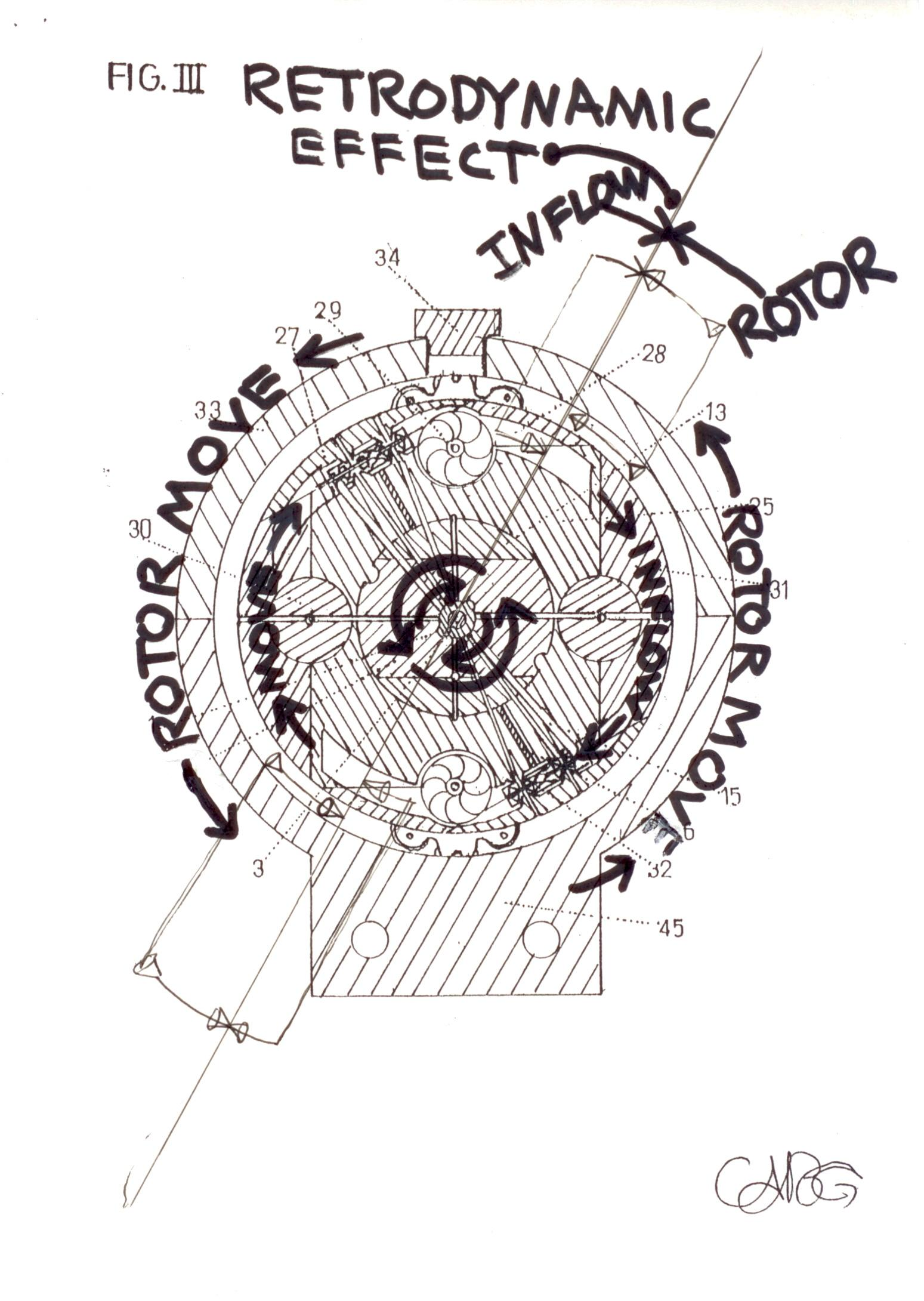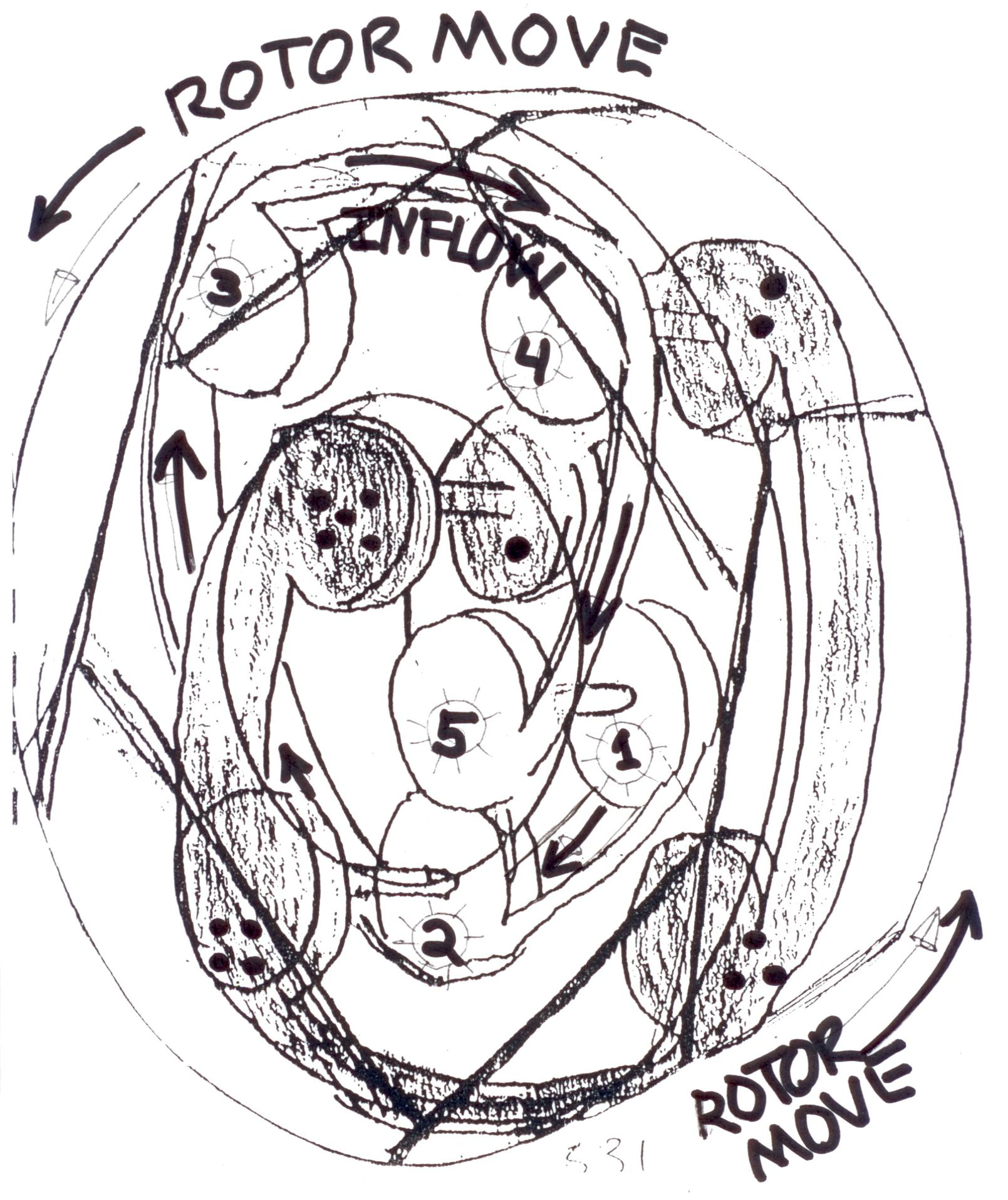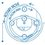# Retrodynamic = Dextrogiro => vs <= Levogiro - Phenomenon EffectRetrodynamic: Dextrogiro vs Levogiro Phenomenon Effect. / Rotor-RPM VS InFlow / front to front; "Collision-Interaction Type" - inflow vs blades-gear-move. Technical unique dynamic innovative motion mode. [Retrodynamic Reaction = When the inflow have more velocity the rotor have more RPM Acceleration, with high (XY Position) Momentum] Which the internal flow (and rotor) duplicate its speed, when activated being in a rotor (and inflow) with [inverse] opposite Turns. The Reaction at front of the action. A very strong Novel torque power concept.

"Changing rotation inside a mass makes it possible to change its inertial properties. It is the equation for a jet motion without rejection of any mass.” Albert Einstein.

The logic of creation of an inertial propulsion system is thus: Any motion is rotation ---- Rotation of a matter generates a space-time Torsion ---Torsion of space - time is described by Ricci torsion --- Ricci torsion is an inertial field-----the rest mass of any object is determined by its inertial field---- operating by fields and forces of inertia inside of mass we can create inertial propulsion system which moves according to the equation [m (t) dv/dt =-vdm/dt].

Newton's Third Law of Motion: III. For every action there is an equal and opposite reaction.Note by Carlos Barrera
2 years, 11 months ago

This discussion board is a place to discuss our Daily Challenges and the math and science related to those challenges. Explanations are more than just a solution — they should explain the steps and thinking strategies that you used to obtain the solution. Comments should further the discussion of math and science.

When posting on Brilliant:

• Use the emojis to react to an explanation, whether you're congratulating a job well done , or just really confused .
• Ask specific questions about the challenge or the steps in somebody's explanation. Well-posed questions can add a lot to the discussion, but posting "I don't understand!" doesn't help anyone.
• Try to contribute something new to the discussion, whether it is an extension, generalization or other idea related to the challenge.

MarkdownAppears as
*italics* or _italics_ italics
**bold** or __bold__ bold
- bulleted- list
• bulleted
• list
1. numbered2. list
1. numbered
2. list
Note: you must add a full line of space before and after lists for them to show up correctly
paragraph 1paragraph 2

paragraph 1

paragraph 2

[example link](https://brilliant.org)example link
> This is a quote
This is a quote
    # I indented these lines
# 4 spaces, and now they show
# up as a code block.

print "hello world"
# I indented these lines
# 4 spaces, and now they show
# up as a code block.

print "hello world"
MathAppears as
Remember to wrap math in $$ ... $$ or $ ... $ to ensure proper formatting.
2 \times 3 $2 \times 3$
2^{34} $2^{34}$
a_{i-1} $a_{i-1}$
\frac{2}{3} $\frac{2}{3}$
\sqrt{2} $\sqrt{2}$
\sum_{i=1}^3 $\sum_{i=1}^3$
\sin \theta $\sin \theta$
\boxed{123} $\boxed{123}$